Math resources Geometry Area

Area of a rectangle

# Area of a rectangle

Here you will learn about finding the area of a rectangle, including finding the area of rectilinear figures, finding missing side lengths, solving area problems involving unit conversion, and solving real-world word problems involving the area of a rectangle.

Students will first learn about area of a rectangle as part of measurement and data in 3rd grade.

## What is the area of a rectangle?

The area of a rectangle is the amount of space inside the rectangle. It is measured in units squared, or square units. ( cm^2, m^2, in^2, etc.)

In order to find the area, you need to use the area formula:

\text { Area }=\text { length} \times \text { width}

For example,

\begin{aligned} \text { Area } & =\text { length } \times \text { width } \\\\ & =7 \times 4 \\\\ & =28 \mathrm{~m}^2 \end{aligned}

You can also use this area formula:

\text { Area }=\text { base} \times \text { height}

Notice it multiplies the same parts of the rectangle as the formula A=l \times w, but uses the terms base and height instead of length and width. Either of these formulas can be used to find the area of a rectangle.

A rectangle is a quadrilateral ( 4 sided shape) where every angle is a right angle (90^{\circ}). Opposite sides of the rectangle are equal length.

The area of a rectangle is calculated by multiplying the length of the rectangle by the width of the rectangle.

The final answer must be in square units. For example, square centimeters (cm^2), square meters (m^2), square feet (ft^2), square inches (in^2), etc.

### What is the area of a rectangle?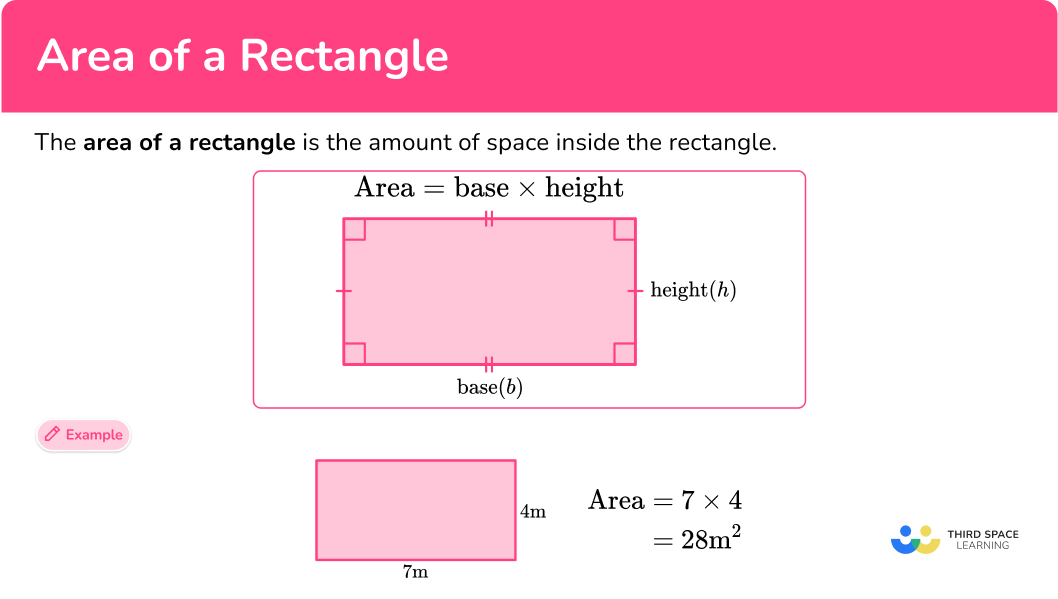## Common Core State Standards

How does this relate to 3rd grade math and 4th grade math?

• Grade 3 – Measurement and Data (3.MD.7)
Relate area to the operations of multiplication and addition.
a. Find the area of a rectangle with whole-number side lengths by tiling it, and
show that the area is the same as would be found by multiplying the side
lengths.
b. Multiply side lengths to find areas of rectangles with whole number side
lengths in the context of solving real world and mathematical problems,
and represent whole-number products as rectangular areas in mathematical
reasoning.
c. Use tiling to show in a concrete case that the area of a rectangle with whole-
number side lengths a and b + c is the sum of a \times b and a \times c. Use area
models to represent the distributive property in mathematical reasoning.
d. Recognize area as additive. Find areas of rectilinear figures by decomposing
them into non-overlapping rectangles and adding the areas of the non-
overlapping parts, applying this technique to solve real world problems.

• Grade 4 – Measurement and Data (4.MD.3)
Apply the area and perimeter formulas for rectangles in real world and mathematical problems. For example, find the width of a rectangular room given the area of the flooring and the length, by viewing the area formula as a multiplication equation with an unknown factor.

## How to find the area of a rectangle

In order to find the area of a rectangle:

1. Identify the length and width of the rectangle.
2. Write down the formula for the area of a rectangle.
3. Substitute the given values and calculate.

## Area of a rectangle examples

### Example 1: finding area given the length and width

Find the area of the rectangle below.

1. Identify the length and width of the rectangle.

Length = 6 \, m

Width = 4 \, m

2Write down the formula for the area of a rectangle.

\text { Area }=\text { length } \times \text { width }

3Substitute the given values and calculate.

\begin{aligned} \text { Area }&=\text { length } \times \text { width } \\\\ \text { Area }&=6 \times 4 \\\\ & =24 \end{aligned}

In this case, you are working with meters so your final answer must be in square meters.

\text { Area }=24 \mathrm{~m}^{2}

### Example 2: finding the area of a rectangle requiring converting units

Find the area of the rectangle below.

Identify the length and width of the rectangle.

Write down the formula for the area of a rectangle.

Substitute the given values and calculate.

### Example 3: real-world word problem

Ms. Crawely is tiling her bathroom floor. The dimensions of the floor are 6 \, m by 4 \, m. Each tile is 50 \, cm by 50 \, cm. How many tiles will she need to cover the bathroom floor?

Identify the length and width of the rectangle.

Write down the formula for the area of a rectangle.

Substitute the given values and calculate.

### Example 4: calculating side length given the area

Find the width of the rectangle below.

Identify the length and width of the rectangle.

Write down the formula for the area of a rectangle.

Substitute the given values and calculate.

### Example 5: area of a rectilinear figure

Find the area of the field below:

Identify the length and width of the rectangle.

Write down the formula for the area of a rectangle.

Substitute the given values and calculate.

### Example 6: word problem

Rectangle ABCD, shown below, has a perimeter of 22 \, m and a side length of 8 \, m. Find the area of the rectangle.

Identify the length and width of the rectangle.

Write down the formula for the area of a rectangle.

Substitute the given values and calculate.

### Teaching tips for area of a rectangle

• Before learning the area of a rectangle formula, allow students to explore area by tiling with square tiles. These concrete manipulatives act as unit squares and will help students build an understanding of area before they begin calculating it.

• Rather than having students practice finding the area of a rectangle on multiple skill worksheets, provide them with a variety of practice problems, activities, and/or projects that have a real-world context. This will deepen their understanding of this skill.

### Easy mistakes to make

• Using incorrect units for the answer
A common error is to forget to include square units in your answer when finding the area of a rectangle.

• Forgetting to convert measures to a common unit
Before using the formula for the area of a rectangle, you need to ensure that the units are the same. If different units are given (for example, length = 4 \, m and width = 3 \, cm ), then you must convert them either both to centimeters or both to meters.

### Practice area of a rectangle questions

1. Find the area of the rectangle below.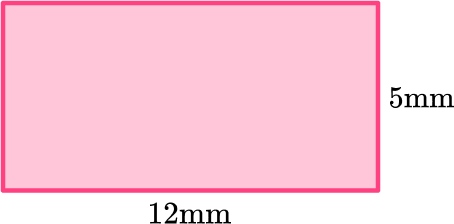60 \, mm17 \, mm^260 \, mm^217 \, mmMultiply the length and width together to get the area of the rectangle.

12 \mathrm{~mm} \times 5 \mathrm{~mm}=60 \mathrm{~mm}^2

2. Find the area of the rectangle below.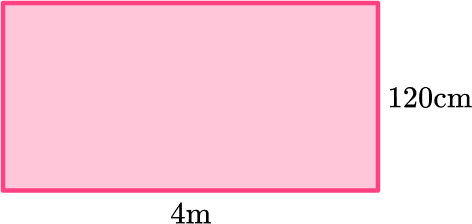480 \, m^2480 \, cm^24.8 \, m^2 or 48 \, 000 \, cm^2124 \, m^2Prior to multiplying the length times the width, convert the length and width to a common unit (both length and width to meters or both to centimeters).

Remember there are 100 centimeters in 1 meter.

3. Mr. Measure is tiling his kitchen floor. The dimensions of the floor are 7 \, m by 5 \, m. Each tile is 20 \, cm by 20 \, cm. How many tiles will he need to cover the bathroom floor?

35 tiles875 tiles400 tiles11 tilesFind the area of the floor by multiplying the length of the rectangle times the width.

Prior to multiplying the length and width for the tile, convert the length and width to a common unit (since you calculated the floor in meters here, you converted tiles to meters).

Remember there are 100 centimeters in 1 meter.

Take the area of the tiles and divide it into the area of the floor to get the number of tiles needed to cover the floor.

4. Find the width of the rectangle below.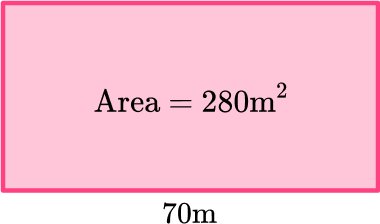4 \, m19600 \, m19 \, 600 \, m^24 \, m^2Using the formula for the area of a rectangle, substitute your given values.

As you are trying to find the width of the rectangle, you need to rearrange the formula dividing the length into the area.

5. Below is a blueprint for a new flower bed. Find the area of the flower bed.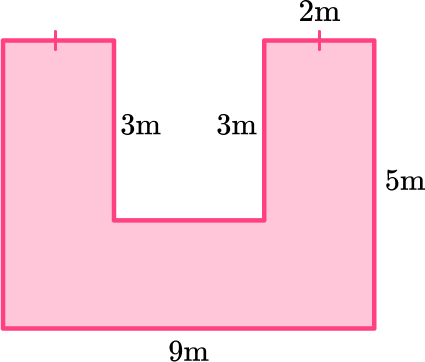57 \, m^222 \, m^245 \, m^230 \, m^2Split the rectilinear figure into 3 rectangles.

Find the missing side length of rectangle C by subtracting 3 from the total length which is 5 \, m.

Add up all the individual areas to get the total area of the rectilinear figure.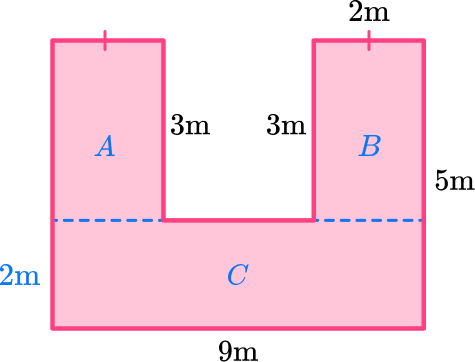Rectangle A

\begin{aligned} \text { Area }&=\text { length } \times \text { width } \\\\ &=3 \times 2 \\\\ &=6 \\\\ \text { Area }&=6 \, m^2 \end{aligned}

Rectangle B

\begin{aligned} \text { Area }&=\text { length } \times \text { width } \\\\ &=3 \times 2 \\\\ &=6 \\\\ \text { Area }&=6 \, m^2 \end{aligned}

Rectangle C

\begin{aligned} \text { Area }&=\text { length } \times \text { width } \\\\ &=9 \times 2 \\\\ &=18 \\\\ \text { Area }&=18 \, m^2 \end{aligned}

\begin{aligned} \text { Total Area }&= 6 \, m^2+6 \, m^2+18 \, m^2 \\\\ & =30 \, m^2 \end{aligned}

6. The perimeter of rectangle WXYZ is 26 \, m. It has a side length of 11 \, m. Find the area of the rectangle.2 \, m2 \, m^222 \, m^222 \, mSince side XY is 11 \, m, side WZ is also 11 \, m. That means the sum of the remaining side lengths must equal 4 \, m.

So side WX and side YZ are each 2 \, m. Then, you multiply 11 \, m by 2 \, m \; (length \times width) to find the area.

## Area of a rectangle FAQs

How do you find the area of a rectangle?

Multiply the length of a rectangle by the width of a rectangle to find its area.

What is the difference between the area of a rectangle and the perimeter of a rectangle?

The area of a rectangle is the amount of space inside the rectangle. It is measured in square units. The perimeter of a rectangle is the distance around the outside of a rectangle, or the sum of the sides of a rectangle. It is measured in any unit of length.

What is the area of a rectangle formula?

The formula for area of a rectangle is Area = length \times width.

## Still stuck?

At Third Space Learning, we specialize in helping teachers and school leaders to provide personalized math support for more of their students through high-quality, online one-on-one math tutoring delivered by subject experts.

Each week, our tutors support thousands of students who are at risk of not meeting their grade-level expectations, and help accelerate their progress and boost their confidence.